# Riemann-Hilbert problem

(Redirected from Riemann–Hilbert problem)
Jump to: navigation, search

linear conjugation problem, Riemann problem, Hilbert problem, Riemann boundary value problem, Riemann–Privalov problem, Hilbert–Privalov problem

One of the main boundary value problems of analytic function theory. It can be stated in the simplest case as follows. Letbe a simple smooth closed contour that splits the plane into a bounded interior domain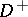and the domain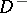complementary to it, containing the point at infinity. Let two functions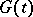andbe given on, satisfying a Hölder condition (-condition), witheverywhere on. It is required to find two functions, analytic in, respectively, continuous up to the contour except for finitely many points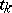, where they may have discontinuities satisfying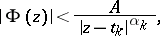and satisfying onthe boundary condition(*)

where the functionis called the coefficient of the problem. The integeris called the index of the coefficientand at the same time the index of the Riemann–Hilbert problem. Suppose that one is looking for a solution satisfying the condition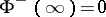. Then for, the homogeneous Riemann–Hilbert problem (that is, when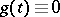) and the non-homogeneous Riemann–Hilbert problem are unconditionally solvable; the solution depends linearly onarbitrary constants and are expressed linearly in terms of a polynomial of degreewith arbitrary coefficients. If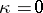, the homogeneous Riemann–Hilbert problem has only the trivial zero solution, and the non-homogeneous problem is solvable unconditionally and uniquely. If, the homogeneous problem has only the trivial solution, and the non-homogeneous problem has a unique solution only if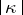solvability conditions are satisfied, which can be expressed linearly in terms of a polynomial with variable coefficients.

The solution of the Riemann–Hilbert problem in all cases is represented in closed form by quadratures in terms of integrals of Cauchy type (cf. Cauchy integral). The boundary values of the unknown functions necessarily satisfy the-condition on the contour. The result listed above can be carried over unchanged to the case of a multiply-connected domainbounded by finitely many simple mutually non-intersecting closed curves. The case of a contour consisting of open curves presents the peculiarity that at the ends of the curves of the contour the solutions of the Riemann–Hilbert problem, depending on the chosen class of solutions, may become infinite or remain bounded. The index depends on the chosen class of solutions. The index in the class of solutions with an admissible infinity at the end of order less than one (an integrable infinity) is equal to one plus the index in the class of solutions bounded at this end. In accordance with this, the number of linearly independent solutions increases by one or the number of solvability conditions decreases by one. A similar situation occurs in the case when the coefficienthas discontinuities of the first kind; the solution at the points of discontinuity behaves in the same way as at the ends of the contour.

In the general case, when the contour consists of finitely many arbitrarily situated closed and open curves, the Riemann–Hilbert problem is solved by the same methods as the simple cases mentioned above, and similar results hold. Some difficulties are presented by the investigation of the solution at the points where several curves of the contour meet.

In the case whenand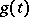are only continuous, but do not satisfy an-condition, the results stated above remain valid, except that here the boundary values of the solutions exist only as the contour is approached along non-tangential paths, and they are not continuous, but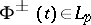for any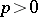; ifis continuous and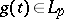, then. The most general assumption for the coefficientunder which the Riemann–Hilbert problem has been solved is that it belongs to the class of measurable functions with an additional condition on the value of the jump of the argument; here also.

Riemann–Hilbert problems with infinite index have been considered, in which simple smooth curves have been chosen for the contours with one or both ends going to infinity. The following cases have been investigated: 1) a polynomial order of growth, when as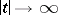the asymptotic equalities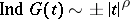are satisfied (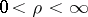for the case one infinite end,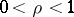for both ends infinite); and 2) a logarithmic order of growth, when as,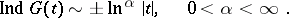At both ends with a positively infinite index the number of linearly independent solutions is infinite and is expressed in terms of an entire function whose form depends on the order of the indices. For a negative infinite index the homogeneous problems has no non-trivial solutions, and the non-homogeneous problem is solvable only if an infinite set of solvability conditions is satisfied. The main difficulty lies in distinguishing the finite solutions.

The solution of the Riemann–Hilbert problem on a Riemann surface, and the equivalent problem on the fundamental domain of an automorphic function belonging to a group of permutations, has been investigated for automorphic functions of this class. The number of solutions or solvability conditions depends on the index, and in certain (singular) cases also on the genus of the surface or on the fundamental domain.

If in condition (*)is a matrix and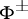andare (-dimensional) vectors, then there arises the Riemann–Hilbert problem for a componentwise-analytic vector. This is significantly more complicated than the scalar caseconsidered above. The investigation is carried out by reduction to a system of integral equations. The number of linearly independent solutions or solvability conditions depends onunknown quantities, called partial indices, the dependence of which on the coefficient matrix has not been established explicitly. An important role is played by the index of the determinant of, called the total index of the problem.

The Riemann–Hilbert problem (for a componentwise-analytic vector) occurred first with B. Riemann (see ) in connection with the solution of the problem of constructing a linear differential equation from a given group of permutations (monodromy group). However, in the approximate form stated above the Riemann–Hilbert problem was first considered by D. Hilbert in 1905 (see ) under less general conditions; the first results (of an alternative character) were obtained by reduction to an integral equation. In 1908 J. Plemelj (see ), using for the first time integrals of Cauchy type, reduced the Riemann–Hilbert vector problem to an integral equation and completely solved the problem of differential equations posed by Riemann. The non-homogeneous Riemann–Hilbert problem (in a somewhat different formulation) was first considered by I.I. Privalov ; his results are concerned mainly with function-theoretical generalizations.

The Riemann–Hilbert problem has many applications. The main ones are in the theory of singular integral equations. Generalizations have been given in various directions: the Riemann–Hilbert problem with a shift or with conjugation, with differentials for generalized analytic functions, and others. The theory of the Riemann–Hilbert problem and its generalizations and applications are more fully reflected in  and .